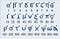How did Ancient Greeks count

We all know very well the roman numeral system, but what about Greeks?

Ancient Greeks developed two different systems: Attic and Jonic.

Attic System

Let's start with the Attic system developed around the 6th-5th century BC. Which used the following symbols:

These symbols (except the first one) came from the first letter of each number in the archaic script: ΠΕΝΤΕ (5), ΔΕΚΑ (10), ΗΕΚΑΤΟΝ (100), ΧΙΛΙΟΙ (1.000), ΜΥΡΙΑΕ (10.000).

So if they wanted to represent the number 7 they would use:

But to make it easier for representing higher numbers, they used a combination of two symbols to represent 50, 500 and 5000, 50000. They were obtained by adding the sign of 10, 100, 1000, 10000 into the sign of 5. E.g. 5000 would be:

Jonic System

Around the 4th-3rd Century BC, they developed the Jonic System, based on powers of 10. It used the alphabet to represent numbers. Each letter of the alphabet had a numeric value. Numbers 1–9 were assigned to the 9 first letters from alpha (α) to theta (θ). Then, each multiple of ten from 10–90 was assigned to the following letters from iota (ι) to koppa* (Ϙ). And finally, the multiples of one hundred from 100–900 were assigned to the following letters from rho (ρ) to sampi* (ϡ).*Note: in this picture appears 3 letters which no longer exist: digamma (ϛ), koppa (Ϙ), and sampi (ϡ).

To represent the number, the numeric values of the letters are represented from highest to lowest and are added together to obtain the total.

An acute accent (ʹ)is placed at the end of the group of letters to distinguish numbers from real words.

To represent numbers from 1000 onwards, a comma is added at the beginning of the letter to indicate that the letter is multiplied by 1000.

The number 0 didn’t exist.

So lets see some examples:

132= ρμβʹ

24= κδʹ

75829= ,ο,εωκθʹ

603= χγʹ

50508= ,νφηʹ

Latin and Ancient Greek lover!

More from María Sánchez

Latin and Ancient Greek lover!